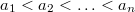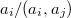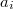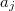﻿

### Note on squarefree integers through a set theoretical property

#### Abstract

In this paper, we show a property of set theory, that in number theory has the following consequence: if$a_{1} < a_{2} < \ldots < a_{n}$ are squarefree integers, then the number of distinct ratios$a_{i}/(a_{i},a_{j})$ is greater than or equal to n, where$(a_{i},a_{j})$ denotes the greatest common divisor of$a_{i}$ and$a_{j}$.

DOI Code: 10.1285/i15900932v19n2p227

Full Text: PDF